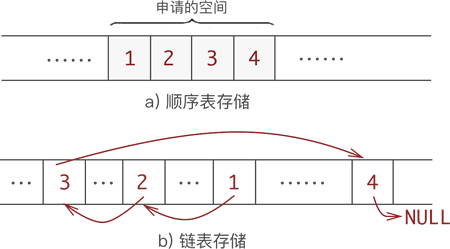# [原创]求质数 之 筛法(C语言描述)

+关注继续查看

## 问题分析

2 3 4 5 6 7 8 9 10 11 12 13 14 15 16 17 18 19 20

2 | 3 5 7 9 11 13 15 17 19

2 3 | 5 7 11 13 17 19

### 筛法的原理：

1. 数字2是素数。
2. 在数字K前，每找到一个素数，都会删除它的倍数，即以它为因子的整数。如果k未被删除，就表示2->k-1都不是k的因子，那k自然就是素数了。

### 算法优化

1. 除余法那篇文章里也介绍了，要找出一个数的因子，其实不需要检查 2→k，只要从 2->sqrt(k)，就可以了。所有，我们筛法里，其实只要筛到sqrt(n)就已经找出所有的素数了，其中n为要搜索的范围。
2. 另外，我们不难发现，每找到一个素数 k，就一次删除 2k, 3k, 4k,..., ik，不免还是有些浪费，因为2k已经在找到素数2的时候删除过了，3k已经在找到素数3的时候删除了。因此，当 i＜k 时，都已经被前面的素数删除过了，只有那些最小的质因子是k的那些数还未被删除过，所有，就可以直接从 k*k 开始删除。
3. 再有，所有的素数中，除了 2 以外，其他的都是奇数，那么，当 i 是奇数的时候，ik 就是奇数，此时 k*k+ik 就是个偶数，偶数已经被2删除了，所有我们就可以以2k为单位删除步长，依次删除 k*k, k*k+2k, k*k+4k, ...。
4. 我们都清楚，在前面一小段范围内，素数是比较集中的，比如 1→100 之间就有25个素数。越到后面就越稀疏。

gab = 4; for (k = 5; k * k <= N; k += gab ^= 6) { ... }

k2 = (6n+1)*(6n+1) = 36n2 + 12n + 1，其中 36n2+12n = 6(6n2+2n) 是6的倍数，所以 k除 6 余 1。

len = k*(k+4)/6*2 - k2/6*2 = (6n+1)*(6n+1+4)/6*2+1 - (6n+1)*(6n+1)/6*2 = (12n2+12n+1) - (12n2+4n) = 8n+1;
stp = k*(k+6)/6*2 - k2/6*2 = 12n+2;

len = 8n+1;
stp = 12n+2;
j = 12n2+4n;

len = 4n+3;
stp = 12n+10;
j = 12n2+20n+8;

5. 由(2)可知，如果每找到一个素数k，能依次只删除以k为最小素数因子的数，那么每个数字就都只被删除一次，那这个筛法就能达到线性的 O(n) 效率了。比如数字 600 = 2*2*3*5*11，其中 2 是它的最小素数因子。那这个数就被 2 删除了。3、5、11虽然都是它的因子，但不做删除它的操作。要实现这种策略，那每找到一个素数 k，那从 k 开始，一次后面未被删除的数字来与 k 相乘，删除它们的积。比如要筛出 2～60 之间的素数：

1. 先列出所有的数。

02 03 04 05 06 07 08 09 10 11 12 13 14 15 ... 50 51 52 53 54 55 56 57 58 59 60

2. 选出序列中的第一个数 2，判定它是素数，然后从 2 开始，依次与剩下的未被删除的数相乘，删除它们的积。即 2*2=4， 2*3=6，2*4=8...。

02 03 04 05 06 07 08 09 10 11 12 13 14 15 ... 50 51 52 53 54 55 56 57 58 59 60
02 | 03 05 07 09 11 13 15 17 19 21 23 25 27 29 31 33 35 37 39 41 43 45 47 49 51 53 55 57 59

3. 去掉 2 后，再选出序列中第一个数 3，判定它是素数，然后从 3 开始，依次与剩下的数相乘，即 3*3=9，3*5=15，3*7=21...

02 | 03 05 07 09 11 13 15 17 19 21 23 25 27 29 31 33 35 37 39 41 43 45 47 49 51 53 55 57 59
02 03 | 05 07 11 13 17 19 23 25 29 31 35 37 41 43 47 49 53 55 59

4. 去掉 3 后，选出最小的数 5，判定它为素数，依次删除 5*5=25，5*7=35，5*11=55，...

02 03 | 05 07 11 13 17 19 23 25 29 31 35 37 41 43 47 49 53 55 59
02 03 05 | 07 11 13 17 19 23 29 31 37 41 43 47 49 53 59

5. 去掉5后，选出最小的数7，为素数，删除7*7=49，...

02 03 05 | 07 11 13 17 19 23 29 31 37 41 43 47 49 53 59
02 03 05 | 07 11 13 17 19 23 29 31 37 41 43 47 53 59

6. 去掉7后，第一个数 11 的平方 121 大于 60，所以结束。剩下的数字全为素数。

02 03 05 07 11 13 17 19 23 29 31 37 41 43 47 53 59 |

## 程序清单

#include <stdlib.h> #include <stdio.h> #include <time.h> #define N 100000000 #define size (N/6*2 + (N%6 == 5? 2: (N%6>0))) int p[size / 32 + 1] = {1}; int creat_prime(void) { int i, j; int len, stp; int c = size + 1; for (i = 1; ((i&~1)<<1) * ((i&~1) + (i>>1) + 1) < size; i++) { if (p[i >> 5] >> (i & 31) & 1) continue; len = (i & 1)? ((i&~1)<<1) + 3: ((i&~1)<<2) + 1; stp = ((i&~1)<<1) + ((i&~1)<<2) + ((i & 1)? 10: 2); j = ((i&~1)<<1) * (((i&~1)>>1) + (i&~1) + 1) + ((i & 1)? ((i&~1)<<3) + 8 + len: len); for (; j < size; j += stp) { if (p[j >> 5] >> (j & 31) & 1 ^ 1) p[j >> 5] |= 1L << (j & 31), --c; if (p[(j-len) >> 5] >> ((j-len) & 31) & 1 ^ 1) p[(j-len) >> 5] |= 1L << ((j-len) & 31), --c; } if (j - len < size && (p[(j-len) >> 5] >> ((j-len) & 31) & 1 ^ 1)) p[(j-len) >> 5] |= 1L << ((j-len) & 31), --c; } return c; } int main(void) { clock_t t = clock(); printf("%d ", creat_prime()); printf("Time: %f ", 1.0 * (clock() - t) / CLOCKS_PER_SEC); return EXIT_SUCCESS; }

## 运行结果

5761455
Time: 0.300000

## 联系方式

|
3月前
|

31 0
|
7月前
|

C语言实现嵌入式状态机简单描述与应用
C语言实现嵌入式状态机简单描述与应用
215 0
|

162 0|

|

1120 0
|